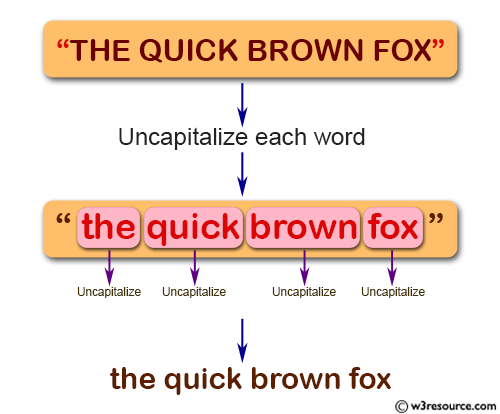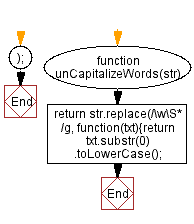# JavaScript: Uncapitalize each word in the string

## JavaScript String: Exercise-42 with Solution

Write a JavaScript function to uncapitalize each word in the string.

Test Data:
console.log(unCapitalizeWords('JS STRING EXERCISES'));
"js string exercises"

Pictorial Presentation:Sample Solution:-

HTML Code:

``````<!DOCTYPE html>
<html>
<meta charset="utf-8">
<title>JavaScript function to uncapitalize each word in the string</title>
<body>

</body>
</html>
```
```

JavaScript Code:

``````function unCapitalizeWords(str)
{
return str.replace(/\w\S*/g, function(txt){return txt.substr(0).toLowerCase();});
}
console.log(unCapitalizeWords('JS STRING EXERCISES'));
```
```

Sample Output:

```js string exercises
```

Flowchart:Live Demo:

See the Pen JavaScript Uncapitalize each word in the string-string-ex-42 by w3resource (@w3resource) on CodePen.

What is the difficulty level of this exercise?

Test your Programming skills with w3resource's quiz.

﻿

## JavaScript: Tips of the Day

Returns the symmetric difference between two arrays, after applying the provided function to each array element of both

Example:

```const tips_symmetricDifference = (x, y, fn) => {
const sA = new Set(x.map(v => fn(v))),
sB = new Set(y.map(v => fn(v)));
return [...x.filter(x => !sB.has(fn(x))), ...y.filter(x => !sA.has(fn(x)))];
};

console.log(tips_symmetricDifference([3.5, 5.5], [5.5, 7.5], Math.floor));
```

Output:

```[3.5, 7.5]
```